In [ ]:
from fastai import *
from fastai.vision import *


The blog can be found at this link.

In [ ]:
# Code for Weight Standardization
class Conv2d(nn.Conv2d):
def __init__(self, in_channels, out_channels, kernel_size, stride=1,
super().__init__(in_channels, out_channels, kernel_size, stride,

def forward(self, x):
weight = self.weight
weight_mean = weight.mean(dim=1, keepdim=True).mean(dim=2,
keepdim=True).mean(dim=3, keepdim=True)
weight = weight - weight_mean
std = weight.view(weight.size(0), -1).std(dim=1).view(-1, 1, 1, 1) + 1e-5
weight = weight / std.expand_as(weight)
return F.conv2d(x, weight, self.bias, self.stride,


### Define model¶

I test with Resnet-18

In [ ]:
class BasicBlock(nn.Module):
expansion = 1
def __init__(self, inplanes, planes, stride=1, downsample=None, groups=1, norm_layer=None, use_ws=False):
super().__init__()
if use_ws:
my_conv = Conv2d
else:
my_conv = nn.Conv2d

if norm_layer is None:
norm_layer = nn.BatchNorm2d
if groups != 1:
raise ValueError('BasicBlock only supports groups=1')
# Both self.conv1 and self.downsample layers downsample the input when stride != 1
self.conv1 = my_conv(inplanes, planes, kernel_size=3, stride=stride, padding=1, groups=groups, bias=False)
self.bn1 = norm_layer(planes)
self.relu = nn.ReLU(inplace=True)
self.conv2 = my_conv(planes, planes, kernel_size=3, padding=1, groups=groups, bias=False)
self.bn2 = norm_layer(planes)
self.downsample = downsample
self.stride = stride

def forward(self, x):
identity = x

out = self.conv1(x)
out = self.bn1(out)
out = self.relu(out)

out = self.conv2(out)
out = self.bn2(out)

if self.downsample is not None:
identity = self.downsample(x)

out += identity
out = self.relu(out)

return out

class ResNet(nn.Module):
def __init__(self, block, layers, use_ws=False, num_classes=1000, zero_init_residual=False,
groups=1, width_per_group=64, norm_layer=None):
super().__init__()
if norm_layer is None:
norm_layer = nn.BatchNorm2d

if use_ws:
self.my_conv = Conv2d
else:
self.my_conv = nn.Conv2d
self.use_ws = use_ws

planes = [int(width_per_group * groups * 2 ** i) for i in range(4)]
self.inplanes = planes
self.conv1 = self.my_conv(3, planes, kernel_size=3, stride=1, padding=0,
bias=False)
self.bn1 = norm_layer(planes)
self.relu = nn.ReLU(inplace=True)

self.layer1 = self._make_layer(block, planes, layers, groups=groups, norm_layer=norm_layer)
self.layer2 = self._make_layer(block, planes, layers, stride=2, groups=groups, norm_layer=norm_layer)
self.layer3 = self._make_layer(block, planes, layers, stride=2, groups=groups, norm_layer=norm_layer)
self.layer4 = self._make_layer(block, planes, layers, stride=2, groups=groups, norm_layer=norm_layer)
self.fc = nn.Linear(planes * block.expansion, num_classes)

for m in self.modules():
if isinstance(m, nn.Conv2d) or isinstance(m, Conv2d):
nn.init.kaiming_normal_(m.weight, mode='fan_out', nonlinearity='relu')
elif isinstance(m, (nn.BatchNorm2d, nn.GroupNorm)):
nn.init.constant_(m.weight, 1)
nn.init.constant_(m.bias, 0)

# Zero-initialize the last BN in each residual branch,
# so that the residual branch starts with zeros, and each residual block behaves like an identity.
# This improves the model by 0.2~0.3% according to https://arxiv.org/abs/1706.02677
if zero_init_residual:
for m in self.modules():
if isinstance(m, Bottleneck):
nn.init.constant_(m.bn3.weight, 0)
elif isinstance(m, BasicBlock):
nn.init.constant_(m.bn2.weight, 0)

def _make_layer(self, block, planes, blocks, stride=1, groups=1, norm_layer=None):
if norm_layer is None:
norm_layer = nn.BatchNorm2d
downsample = None
if stride != 1 or self.inplanes != planes * block.expansion:
downsample = nn.Sequential(
self.my_conv(self.inplanes, planes * block.expansion, kernel_size=1, stride=stride, bias=False),
norm_layer(planes * block.expansion),
)

layers = []
layers.append(block(self.inplanes, planes, stride, downsample, groups, norm_layer, use_ws=self.use_ws))
self.inplanes = planes * block.expansion
for _ in range(1, blocks):
layers.append(block(self.inplanes, planes, groups=groups, norm_layer=norm_layer, use_ws=self.use_ws))

return nn.Sequential(*layers)

def forward(self, x):
x = self.conv1(x)
x = self.bn1(x)
x = self.relu(x)

x = self.layer1(x)
x = self.layer2(x)
x = self.layer3(x)
x = self.layer4(x)

x = self.avgpool(x)
x = x.view(x.size(0), -1)
x = self.fc(x)

return x

In [ ]:
resnet18 = ResNet(BasicBlock, [2, 2, 2, 2], num_classes=10)
resnet18_ws = ResNet(BasicBlock, [2, 2, 2, 2], num_classes=10, use_ws=True)

# Save state dict, so we have same model weights
state_dict = resnet18.state_dict()

def get_models():
resnet18 = ResNet(BasicBlock, [2, 2, 2, 2], num_classes=10)
resnet18_ws = ResNet(BasicBlock, [2, 2, 2, 2], num_classes=10, use_ws=True)

# Save state dict, so we have same model weights
state_dict = resnet18.state_dict()

return resnet18, resnet18_ws


In [ ]:
# For the data I use CIFAR-10
path = Path('/home/kushaj/Desktop/Data/cifar10/')
src = (ImageList.from_folder(path)
.split_by_folder()
.label_from_folder())

In [ ]:
data = (src.transform(size=(32, 32))
.databunch(path, 64)
.normalize(cifar_stats))


## Start experimentation¶

In [ ]:
# The paper proved that Weight standardization smoothened the loss surface.
# If that is true then we should be able to train our model with higher
# learning rate. So we test that now using lr_find

In [ ]:
learn = Learner(data, resnet18)
learn_ws = Learner(data, resnet18_ws)

In [ ]:
# First I test for model with only Batch Norm
learn.lr_find()

LR Finder is complete, type {learner_name}.recorder.plot() to see the graph.

In [ ]:
lr_find = learn.recorder.plot(return_fig=True)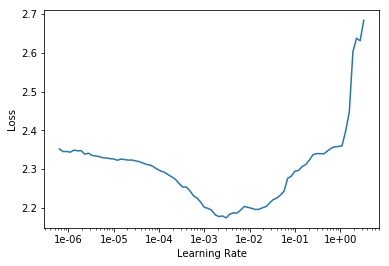In [ ]:
learn_ws.lr_find()

LR Finder is complete, type {learner_name}.recorder.plot() to see the graph.

In [ ]:
lr_find_ws = learn_ws.recorder.plot(return_fig=True)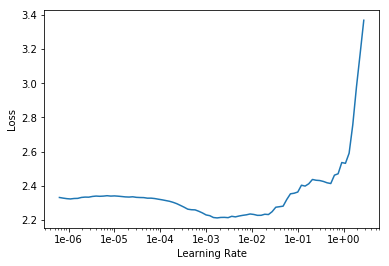In [ ]:
# There is some reason to believe that we can train at higher learning rate
# but the proof is not much significant.

# So how about we train them for some epochs and see which gives smalle loss.

In [ ]:
learn.fit_one_cycle(3, max_lr=0.0005)

Total time: 09:15

epoch train_loss valid_loss time
0 1.073306 1.095837 03:04
1 0.667810 0.688976 03:05
2 0.389454 0.508198 03:05
In [ ]:
# For now let's test with the same learning rate
learn_ws.fit_one_cycle(3, max_lr=0.0005)

Total time: 10:31

epoch train_loss valid_loss time
0 1.029879 1.033030 03:28
1 0.639364 0.666070 03:27
2 0.367526 0.504515 03:34

Let's try out for smaller batch size like 2

In [ ]:
data = (src.transform(size=(32, 32))
.databunch(path, 2)
.normalize(cifar_stats))

resnet18_micro, resnet18_ws_micro = get_models()

learn_micro = Learner(data, resnet18_micro)
learn_ws_micro = Learner(data, resnet18_ws_micro)

In [ ]:
learn_micro.lr_find()

LR Finder is complete, type {learner_name}.recorder.plot() to see the graph.

In [ ]:
learn_micro.recorder.plot()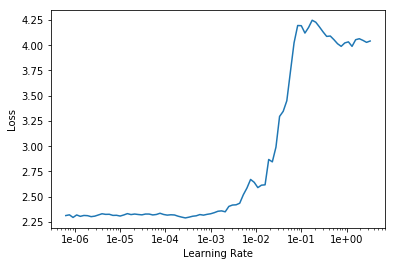In [ ]:
learn_ws_micro.lr_find()

LR Finder is complete, type {learner_name}.recorder.plot() to see the graph.

In [ ]:
learn_ws_micro.recorder.plot()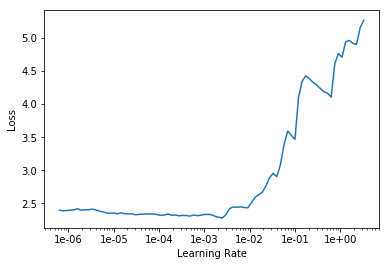In [ ]:
# Here the only difference that I could notice is, in case of learn_micro
# the learn diverged more quickly, than in case of learn_ws_micro. This
# fact supports the idea presented in the paper, as the only reason the loss
# did not diverge in the second case was, because Weight Standardization made
# the loss surface smoother.

In [ ]:
learn_micro.fit_one_cycle(3, max_lr=0.00005)

Total time: 55:13

epoch train_loss valid_loss time
0 1.373090 1.246792 18:28
1 0.918476 0.802182 18:21
2 0.550664 0.695172 18:24
In [ ]:
learn_ws_micro.fit_one_cycle(3, max_lr=0.00005)

Total time: 1:36:51

epoch train_loss valid_loss time
0 1.307534 1.201807 31:50
1 0.835780 0.793317 31:37
2 0.665657 0.670581 33:23
In [ ]:
resnet18_micro, resnet18_ws_micro = get_models()
learn_ws_micro2 = Learner(data, resnet18_ws_micro)

In [ ]:
learn_ws_micro2.fit_one_cycle(2, max_lr=0.001)

Total time: 1:04:50

epoch train_loss valid_loss time
0 1.033272 0.985062 33:18
1 0.575197 0.644893 31:32
In [ ]:
learn_micro2 = Learner(data, resnet18_micro)

In [ ]:
learn_micro2.fit_one_cycle(2, max_lr=0.001)

Total time: 37:23

epoch train_loss valid_loss time
0 1.056560 0.948750 18:56
1 0.698630 0.677414 18:27
In [ ]:
data = (src.transform(size=(32, 32))
.databunch(path, 256)
.normalize(cifar_stats))

resnet18_large, resnet18_ws_large = get_models()
learner_large = Learner(data, resnet18_large)
learner_ws_large = Learner(data, resnet18_ws_large)

In [ ]:
learner_large.lr_find()

LR Finder is complete, type {learner_name}.recorder.plot() to see the graph.

In [ ]:
learner_large.recorder.plot()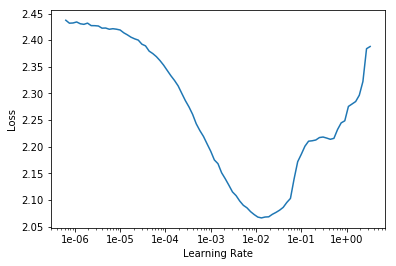In [ ]:
learner_ws_large.lr_find()

LR Finder is complete, type {learner_name}.recorder.plot() to see the graph.

In [ ]:
learner_ws_large.recorder.plot()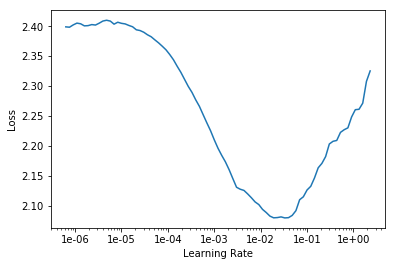In [ ]:
learner_large.fit_one_cycle(10, max_lr=1e-2)

Total time: 29:12

epoch train_loss valid_loss time
0 1.147950 1.410681 02:54
1 0.889552 1.472751 02:55
2 0.707213 0.761191 02:55
3 0.567178 0.725678 02:55
4 0.431706 0.672626 02:55
5 0.284887 0.563302 02:55
6 0.143974 0.581366 02:54
7 0.040767 0.668141 02:54
8 0.006895 0.654571 02:55
9 0.001625 0.663592 02:54
In [ ]:
learner_ws_large.fit_one_cycle(10, max_lr=5e-2)

60.00% [6/10 18:03<12:02]
epoch train_loss valid_loss time
0 1.192142 1.239801 03:00
1 0.894016 0.900090 03:00
2 0.733448 0.888460 03:00
3 0.617678 0.726223 03:00
4 0.514812 0.623703 03:00
5 0.372382 0.580618 03:00

Interrupted
---------------------------------------------------------------------------
KeyboardInterrupt                         Traceback (most recent call last)
<ipython-input-14-8d70bb79369e> in <module>
----> 1 learner_ws_large.fit_one_cycle(10, max_lr=5e-2)

~/anaconda3/envs/PyTorch/lib/python3.7/site-packages/fastai/train.py in fit_one_cycle(learn, cyc_len, max_lr, moms, div_factor, pct_start, final_div, wd, callbacks, tot_epochs, start_epoch)
20     callbacks.append(OneCycleScheduler(learn, max_lr, moms=moms, div_factor=div_factor, pct_start=pct_start,
21                                        final_div=final_div, tot_epochs=tot_epochs, start_epoch=start_epoch))
---> 22     learn.fit(cyc_len, max_lr, wd=wd, callbacks=callbacks)
23
24 def lr_find(learn:Learner, start_lr:Floats=1e-7, end_lr:Floats=10, num_it:int=100, stop_div:bool=True, wd:float=None):

~/anaconda3/envs/PyTorch/lib/python3.7/site-packages/fastai/basic_train.py in fit(self, epochs, lr, wd, callbacks)
189         if defaults.extra_callbacks is not None: callbacks += defaults.extra_callbacks
190         fit(epochs, self.model, self.loss_func, opt=self.opt, data=self.data, metrics=self.metrics,
--> 191             callbacks=self.callbacks+callbacks)
192
193     def create_opt(self, lr:Floats, wd:Floats=0.)->None:

~/anaconda3/envs/PyTorch/lib/python3.7/site-packages/fastai/basic_train.py in fit(epochs, model, loss_func, opt, data, callbacks, metrics)
92             for xb,yb in progress_bar(data.train_dl, parent=pbar):
93                 xb, yb = cb_handler.on_batch_begin(xb, yb)
---> 94                 loss = loss_batch(model, xb, yb, loss_func, opt, cb_handler)
95                 if cb_handler.on_batch_end(loss): break
96

~/anaconda3/envs/PyTorch/lib/python3.7/site-packages/fastai/basic_train.py in loss_batch(model, xb, yb, loss_func, opt, cb_handler)
30
31     if opt is not None:
---> 32         loss,skip_bwd = cb_handler.on_backward_begin(loss)
33         if not skip_bwd:                     loss.backward()
34         if not cb_handler.on_backward_end(): opt.step()

~/anaconda3/envs/PyTorch/lib/python3.7/site-packages/fastai/callback.py in on_backward_begin(self, loss)
272     def on_backward_begin(self, loss:Tensor)->None:
273         "Handle gradient calculation on loss."
KeyboardInterrupt: